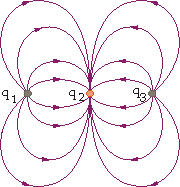# Calculate Charge from Electric Field Diagram?

## Homework Statement

The diagram shows the electric field lines surrounding three electric charges. Assume that q1 + q2 = -2.43 µC.

A) Calculate q2.

B) Calculate q1.

C) Calculate q3.## Homework Equations

|Fe|= (k*|q1|*|q2|)/r^2
|E|=(k*|Q|)/r^2

k = 8.99*10^9 (N*m^2)/C^2
Fe = Electrostatic force between a pair of charges
r = charge separation
q1, q2 = charge magnitudes
E = electric field
Q = charged object

## The Attempt at a Solution

I am having a very hard time with this question. I'm not sure where to start. Based on the diagram I assume that q1 and q3 will be equal in charge and magnitude but am not sure where to go from here.

Any help would be greatly appreciated!

Have you learned Gauss's Law/Maxwell's first equation yet? This problem is essentially that. It involves the fact that electric field lines begin on positive charges, and end on negative charges. So the number of electric field lines around a certain charge distribution is proportional to the net amount of charge in that distribution. If you look at the diagram, you have twice as many field lines entering $q_{2}$ as you have leaving $q_{1}$ and $q_{3}$. Using that, what can you determine about the signs and magnitudes of the charges?